Courses

# Revision Notes - Methods of Enquiry in Psychology Humanities/Arts Notes | EduRev

## Humanities/Arts : Revision Notes - Methods of Enquiry in Psychology Humanities/Arts Notes | EduRev

The document Revision Notes - Methods of Enquiry in Psychology Humanities/Arts Notes | EduRev is a part of the Humanities/Arts Course Psychology Class 11.
All you need of Humanities/Arts at this link: Humanities/Arts

UNIT 2

WHAT IS STATISTICS?

Researchers deal with a large amount of data and have to draw dependable conclusions on the basis of data collected for the purpose.  Statistics help the researchers in making sense of the enormous amount of data.  Let us first understand the term statistics.  Technically “statistics” is that branch of mathematics which deals with numerical data. Researchers are interested in variables.  Variables refer to some aspect of a person, an object or environment that can be measured and whose value can change from one observation to the other.  Statistics deals with description, summarising and representation of data. The inferential statistics helps to draw conclusions from data.  The process of measurement involves use of rules to assign a number to a specific observation of a variable.  Psychologists use four levels of scales: Nominal, Ordinal, Interval, and Ratio.  Nominal scale is at the lowest level and ratio the highest.  In general higher we go up the scale type, more information is contained in the scale.

GRAPHICAL REPRESENTATION OF DATA

After collecting data, the next step is to organize the data to get a quick overview of the same.  Graphical representation helps us in achieving this objective.  It is a part of the descriptive statistics through which we organize and summarise the data.  The outcome is visually presented that makes it easy to see pertinent features of the data.  Such presentations are called graphs.

There are different kinds of graphs.  However, here we shall consider only the Bar Diagram, the Frequency Polygon, and the Histogram.  These graphs have much in common, especially the frequency polygon and histogram, though, they look different.

Basic Procedures

Graphed frequency distributions generally have two axes: horizontal and vertical. The horizontal axis is called X-axis or abscissa and the vertical axis the Y-axis or ordinate.  It is customary to represent the independent variable on the X-axis and dependent variable on the Y-axis. The intersection of the two axes represents the origin or the zero point on the axis.  However, if the initial score (or midpoint of the class interval) of a data to be represented on the graph is away from zero (e.g. midpoint 142 in table 1), we break the horizontal line (axis) to indicate that the portion of the scale is missing.

To make the graph look symmetrical and balanced, it is customary to keep the height of the distribution about three-quarters of the width (height 75 pc of the width).  Some trial and error may be necessary to create graph suitable in size and convenient in scale.  The graph should be given clear and suitable caption with figure number and labels on both the axes.  The caption of a graph is written below the graph with a suitable figure number.

BAR DIAGRAM

The bar diagram represents distribution of categorical data, qualitative categories on a nominal or ordinal scale of measurement.  If the data are on a nominal scale the categories to be represented by the bars on x-axis could be in any meaningful order.  However, if data are on ordinal scale of measurement, the categories should be arranged in order of rank (e.g. students of IX, X, XI, XII).  It is very similar to a histogram (to be taken up little later) in shape.  It is constructed in the same manner except, in the bar diagram, there is space in between the bars or rectangles, which suggests the essential discontinuity of the categories on the X-axis.  The bars could be drawn vertically or horizontally.

Let us explain the procedure of constructing a bar diagram.  Suppose an experimenter is interested in studying the effect of imagery practice on motor learning.  He wants to answer the question: If one practices a given task in imagery how will it affect performance? The experimenter selects two groups of participants randomly.  To one group, he assigns the task to be practiced in imagery and the other group serves as a control.  The task to learn is typewriting.  Twenty trials of imagery practice are given to the experimental group and none to the control group.  The dependent variable constitutes number of errors in typing some material in a given duration of time.  The outcome of the experiment is presented graphically (bar diagram) in fig.1.

It may be noted in Fig.1 that the two bars are separated on the X-axis as the variable represented on the X-axis, the experimental group and control group, is discrete. Another frequently used graph for categorical data is the pie chart.  Unlike the bar diagram, pie charts always use relative frequencies. That is, total area in any pie (circle) is divided into slices representing percent frequency of the total area (100 per cent).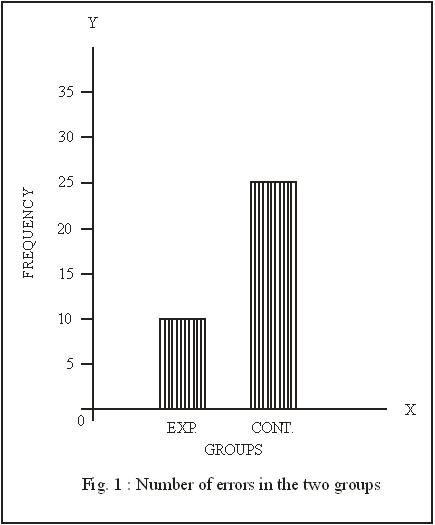FREQUENCY POLYGON

Before you learn to prepare frequency polygon, you should learn how to prepare a frequency distribution from the raw data.

a. Frequency distribution is an orderly arrangement of scores indicating the frequency of each score as shown in table 1.

The ungrouped 50 scores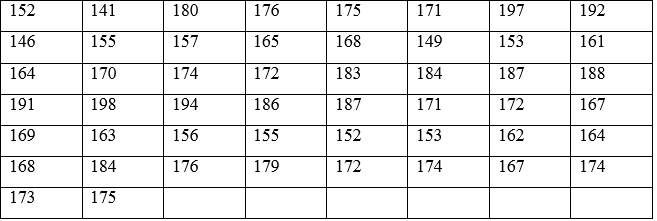Highest score : 198 Lowest score : 141.

b. Constructing a frequency distribution – Before drawing a frequency polygon, we have to first translate a set of raw scores into a frequency distribution.  The procedure of preparing a frequency distribution is given below:

1. Find the lowest and the highest scores in the set of scores.  In the set of scores presented above, the lowest and the highest scores are 141 and 198 respectively.  The range in the scores is 198-141=57.

2. We generally create between 10 to 20 class intervals, and the number of class-intervals will depend upon the interval width (i) we choose.  Interval width, for practical reasons is kept an odd number (so that the mid point representing the class-interval is a whole number).  Here, if we decide to have an i=5, the number of class intervals shall be 57 / 5 (range/i) i.e. 12, which is very much within the convenient range.

3. Next, we must determine the starting point of the bottom class-interval.  The lowest score is 141, thus the lowest interval could be 141 -144 or 140 -144.  We can select 140-144 because 140 is a multiple of our interval width of 5.  This gives us the set of class-intervals shown in table 1.

4. Next, tally the raw scores one by one against the class-intervals.  Then convert the tables into frequencies (f) as shown in the last column of table 1.  Confirm that total of f is equal to n if the distribution is considered sub-sample; or N if it is total sample or total observations.

Frequency Polygon is a line figure used to represent data from a frequency distribution.  The frequency polygon (Greek word meaning many angles) is a series of connected points above the midpoint of each class interval. Each point is at a height equal to the frequency (f) of scores in that interval.  The steps involved in constructing a frequency polygon are:-

1. Prepare a frequency distribution in tabular form.
2. Decide on a suitable scale for X-axis and Y-axis (as explained earlier).
3. Label the midpoints of class interval along the X-axis.
4. Place a point above the midpoint of each class interval at a height equal to the frequency value of the scores in that interval.
5. Connect the points with a straight line.
6. After joining the points bring the polygon down to the horizontal axis (x-axis) at both ends.  One point before the midpoint in the beginning and one point after the last midpoint.

The data together with frequency distribution is presented in Table 1 and frequency polygon is shown in Fig.2.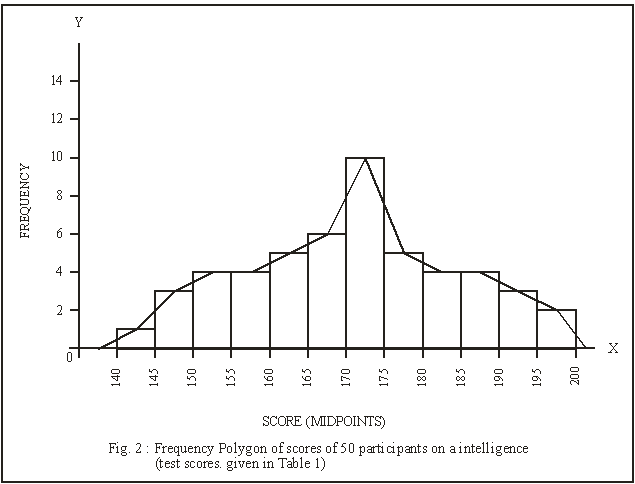Table 1 Frequency Distribution of Scores of students on an Intelligence Test (N=50)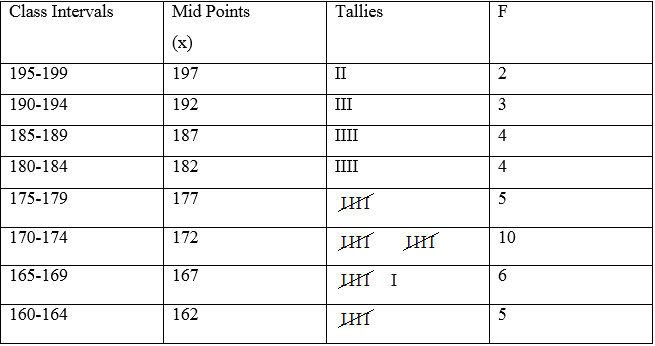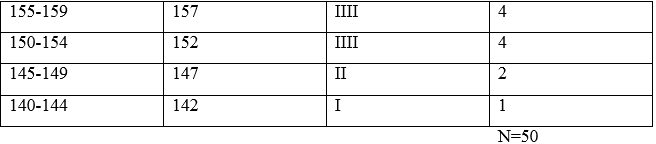Histogram

It is a bar graph that presents data from frequency distribution.  Both polygon and histogram are prepared when data are either on interval or ratio scale.  Both depict the same distribution and you can superimpose one upon the other.  On the same set of data (see Figure 3) and both tell the same story.  However, a polygon is preferred for grouped frequency distribution and histogram in case of ungrouped frequency distribution of a discrete variable or with data treated as discrete variable.  In the frequency polygon all the scores within a given interval are represented by the mid-point of that interval, whereas, in a histogram the scores are assumed to be spread uniformly over the entire interval.  Within each interval of a histogram the frequency is shown by a rectangle, the base being the length of the class interval and the height having frequency within that interval.

Histogram differs from the bar diagram on two counts.  One, histogram is prepared from a data set that is on a continuous series.  Two, the data are obtained on either interval or ratio scale.

In Fig.3 a histogram is prepared from the frequency distribution of scores given in Table 1 and a polygon superimposed to demonstrate the similarity and differences between the two.

The first interval in the histogram actually begins at 139.5, the exact lower limit of the interval and ends at 144.5, the exact upper limit of the interval.  However, we start the first interval of 140 and second at 145, third at 150, and so on.

The frequency of 1 on 140-144 is represented by a rectangle, the base of which is the length of the interval (140-145) and height of which is one unit up on the Y-axis.  Similarly, the frequency of 2 on the next interval is represented by a rectangle one interval long (145-149) and 2 Y units high.  The heights of the other rectangles will vary with the frequencies of the intervals.  Each interval in a histogram is represented by a separate rectangle.  The rise and fall of the rectangles increases or decreases depending on the number of scores for various intervals.  Note, the bars or rectangles are joined together, whereas in the bar diagram they are not.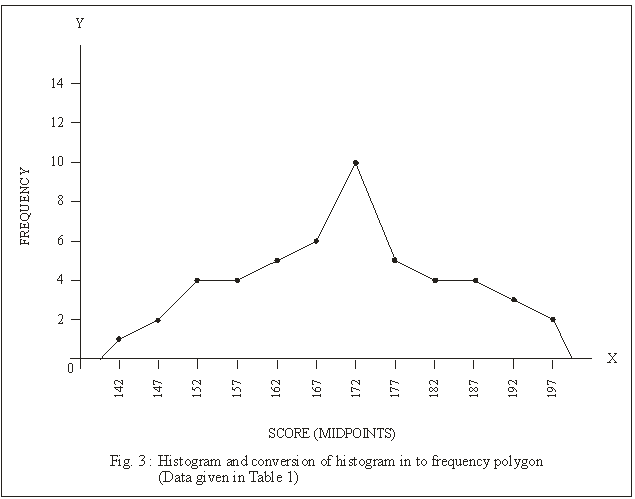As in a frequency polygon, the total frequency (N) is represented by the area of the histogram.  The frequency polygon can be constructed on the same graph by joining the midpoints of each rectangle, as shown in Fig.3.  It may be noted that frequency polygon is less precise than the histogram.  However, if we have to compare two or more distributions, frequency polygons on the same axis are more revealing as compared to histograms.

Recapitulation

After collecting data, the next step is to organize the data to get a quick overview of the entire data.  Graphical representation helps in achieving this objective.  To this end three different kinds of graphs are frequently used : Bar Diagram, Frequency Polygon, and Histogram.  Bar diagram is very similar to a histogram in shape.  However, the bar diagram is used when there is discontinuity between the various categories and space is kept in between the rectangles because the variables represented on the x-axis is discrete.  On the other hand histogram is constructed from data that are on an interval or ratio scales and only when the data are on a continuous series.  Frequency polygon can be constructed on the histogram, by joining the midpoints of each rectangle of the histogram.

MEASURES OF CENTRAL TENDENCY

Suppose that the Principal of your school is interested in knowing how students of psychology in her school compare to students of a nationally renowned school.  She would like to compare the psychology result of the two schools.  The average scores of the two schools can be compared for the purpose.  Measures of this kind are called measures of central tendency.  The purpose is to provide a single summary figure that best describes the central location of the observations or data.  The central tendency of a distribution is the score value near the centre of the distribution.  It represents the basic or central trend in the data.

A measure of central tendency helps simplify comparison of two or more groups.  For example, we have two groups created randomly from a specific population, one group is randomly assigned to treatment condition (Experimental group) and the second is not given any treatment (Control group).  Both the groups are observed on dependent variable after the treatment.  In order to study the effect of treatment the average performance of the two groups needs to be compared.  Later, in this chapter you will discover that we need to know more about the dispersion of scores in the group than just comparing them on some group average.  There are three commonly used measures of central tendency:  Arithmetic Mean, Median, and Mode.  Let us learn about each of these indices and their computation.

The Arithmetic Mean : The arithmetic mean or for brevity mean, is the sum of all the scores in a distribution divided by the total number of scores.  This is also sometimes called average.  We generally do not use the term average because the term is also used for other measures of central tendency.  (We call the men as arithmetic mean because in statistics we also use geometric and harmonic means).

Let us get acquainted with some symbols that we use in calculating central tendencies.

∑          Add

N         The total number of observations in study (N=n1+n2….)

n          The number of observations in each of the subgroups.

X         Raw Scores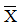Mean of the sample

µ          Mean of the population

Calculation of Mean from un-grouped Data  - Let us take up an example to demonstrate the calculation of mean from the ungrouped data obtained from 10 participants as given below.

X: 8, 7, 3, 9, 4, 4, 5, 6, 8, 8

∑ X=8+7+3+9+4+4+5+6+8+8 =62

Mean ==   ∑X/N = 62/10 = 6.2

Calculation of Mean from Grouped Data

When the data are large, we convert it into frequency distribution by arranging the scores into class intervals, as shown in Table 1.  Let us work out mean from the data grouped into frequency distribution.  The calculation of mean has been given in Table 2.  For grouped data the formula for calculating mean is: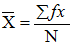Where: f           frequency

X         the mid-point of the class-interval

N         the total number of observations

∑ ƒx   is the sum of the midpoints weighted by their frequencies.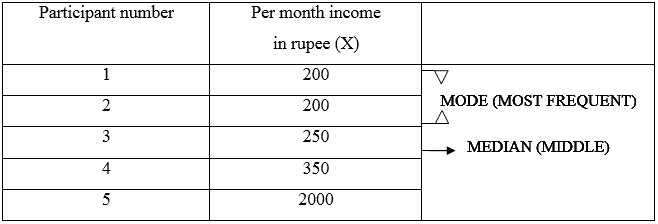∑ X : 3,000 ÷ 5 = 600  MEAN (ARITHMETIC MEAN)

The three measures of central tendency. Generally, the mean is the best index of central tendency, but in this instance the median is more informative.

In this Table the mid points (X) are given against each class-interval.  The X values are multiplied by the respective f to obtain fX, as presented in the last column of the table.  All the fX values are added to get ∑fx.  Finally, ∑fX value is divided by N which is 50.  The mean value comes to 170.7.  This mean has been calculated by the direct method.

The Median : The median is the score value that divides the distribution into halves.  It is such a value that half of the scores in the distribution fall below it and half of them fall above it.

Calculation of Median from Ungrouped Data :  When the scores are not grouped into class intervals in a tablular form, we arrange the scores in the ascending order as given below:

1, 3, 5, 6, 8, 10, 11

When the n is an odd number, the middle score becomes the median.  In the above problem 6 is the median.  The score 6 has an equal number of scores below and above it.  You can observe that there are 3 scores above it and 3 below it.

When the n is even number of scores, there is no middle score, so the median is taken as the point halfway between the two scores.  Let us consider an example.  Suppose, there are 8 students in a class and they get following scores on a test.

0, 3, 5, 6, 7, 10, 11, 12

Table 2  Calculation of Mean from the Grouped data (N=50)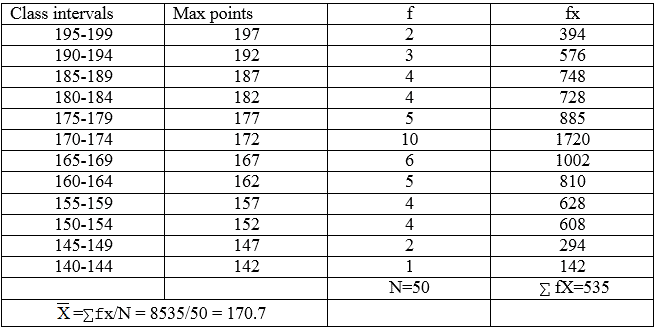The median in the above example is the average of the two middle scores 6 and 7 (6+7/2).

Calculation of Median from Grouped Data  :  The formula for calculating the median when the data are grouped in class intervals is:

Median =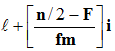Where: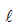=  exact lower limit of the class interval within which the median lies

n/2= one half of the total number of scores

F = sum of the scores of f of all class intervals below l.

fm = frequency (number of scores) within the interval upon which the median falls.

i = size of class interval

Median is a point which divides the scores into two equal halves.  In the above example there should be 25 scores above the median and 25 below.  If we start adding the frequencies (f) from below we discover that 25 lies in the class-interval 170-174, mark the f as indicated in Table 3.  Below the f of 10 the total of frequencies is 22.  The lower limit of the class interval in which the median lies, is 169.5.

Table 3 Calculation of Median from Grouped Data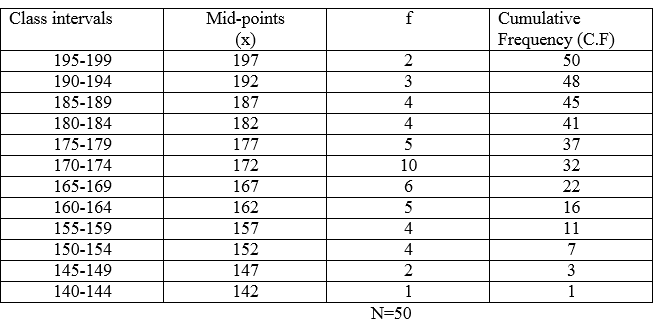Let us apply the Formula to derive Median :

Here :=169.5

n/2       =50/2=25

F          =22

fm       =10

i           =5

:  Median        = 169.5 + (25 – 22/ 10) x 5

=169.5 +1.5 = 171.00

We can also calculate the median by proceeding downwards, from the top.  Let us see how we can work out from the opposite direction.

The median lies in the class interval 170-174 having f of 10.  From top start adding the frequencies till we reach the value 25.  The upper 5 frequencies add upto 18.  So, we require 7 points to make it 25.  to be more precise we need 7 points from 10 to make it to 25.  Therefore, 7 /10x5 = 3.5 should be subtracted from the actual upper limit (174.5) of the class interval in which the median lies.  Therefore, 174.5 – 3.5 = 171.00.  Note, the difference in calculation in proceeding from two different ends of the class interval.

The Mode : The mode (or Mo for brevity), is the score value (or class interval) with the highest frequency.  In an ungrouped data the mode is that single score which occurs in a distribution of scores most frequently.

Calculating Mode from Ungrouped Data :

Consider the following scores of a group of 11 students on a class test of mathematics (arranged in ascending order): µ   £

3, 5, 5, 6, 7, 7, 8, 8, 8, 9, 10

The mode in the above data is 8 because it occurs most frequently, 3 times, in the data.The great advantage of mode, compared to mean and median is that it can be computed for any type of data – obtained through nominal, ordinal, interval, or ratio percentiles. On the other hand, the greatest disadvantage is that it ignores much information available in the data.

Calculating Mode from Grouped data :

A common meaning of mode is ‘fashionable’ and it has the same implication in statistics.  In the frequency distribution given in Table 3 the class interval 170-174 contains the largest frequency (f=10) and 172 being the midpoint is the mode.

When to Use the Mean, Median, and Mode

The Mean is used when :

1. The measure of central value having maximum stability is required.
2. The scores symmetrically fall around a central point i.e. the distribution of scores conform normal distribution.
3. Measures of dispersion, such as standard deviation, are to be calculated.

The Median is used when :

1. The exact 50 per cent point or the midpoint of the distribution is required
2. Extreme scores are likely to affect the mean.  The median is not affected by the extreme scores.
3. Position of an individual score is to be found in terms of its percentage distance from the midpoint of the distribution.

The Mode is used when –

1. Quick and approximate measure of central point of the distribution is required.
2. The measure of the central value is required to denote the most typical characteristic of the group.
Offer running on EduRev: Apply code STAYHOME200 to get INR 200 off on our premium plan EduRev Infinity!

26 docs|2 tests

,

,

,

,

,

,

,

,

,

,

,

,

,

,

,

,

,

,

,

,

,

;